Shortcuts

# Double Backward with Custom Functions¶

It is sometimes useful to run backwards twice through backward graph, for example to compute higher-order gradients. It takes an understanding of autograd and some care to support double backwards, however. Functions that support performing backward a single time are not necessarily equipped to support double backward. In this tutorial we show how to write a custom autograd function that supports double backward, and point out some things to look out for.

When writing a custom autograd function to backward through twice, it is important to know when operations performed in a custom function are recorded by autograd, when they aren’t, and most importantly, how save_for_backward works with all of this.

Custom functions implicitly affects grad mode in two ways:

• During forward, autograd does not record any the graph for any operations performed within the forward function. When forward completes, the backward function of the custom function becomes the grad_fn of each of the forward’s outputs

• During backward, autograd records the computation graph used to compute the backward pass if create_graph is specified

Next, to understand how save_for_backward interacts with the above, we can explore a couple examples:

## Saving the Inputs¶

Consider this simple squaring function. It saves an input tensor for backward. Double backward works automatically when autograd is able to record operations in the backward pass, so there is usually nothing to worry about when we save an input for backward as the input should have grad_fn if it is a function of any tensor that requires grad. This allows the gradients to be properly propagated.

import torch

@staticmethod
def forward(ctx, x):
# Because we are saving one of the inputs use save_for_backward
# Save non-tensors and non-inputs/non-outputs directly on ctx
ctx.save_for_backward(x)
return x**2

@staticmethod
# A function support double backward automatically if autograd
# is able to record the computations performed in backward
x, = ctx.saved_tensors
return grad_out * 2 * x

# Use double precision because finite differencing method magnifies errors
x = torch.rand(3, 3, requires_grad=True, dtype=torch.double)
# Use gradcheck to verify second-order derivatives


We can use torchviz to visualize the graph to see why this works

import torchviz

out = Square.apply(x)


We can see that the gradient wrt to x, is itself a function of x (dout/dx = 2x) And the graph of this function has been properly constructed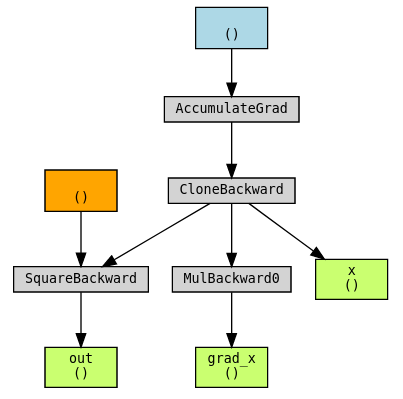## Saving the Outputs¶

A slight variation on the previous example is to save an output instead of input. The mechanics are similar because outputs are also associated with a grad_fn.

class Exp(torch.autograd.Function):
# Simple case where everything goes well
@staticmethod
def forward(ctx, x):
# This time we save the output
result = torch.exp(x)
# Note that we should use save_for_backward here when
# the tensor saved is an ouptut (or an input).
ctx.save_for_backward(result)
return result

@staticmethod
result, = ctx.saved_tensors



Use torchviz to visualize the graph:

out = Exp.apply(x)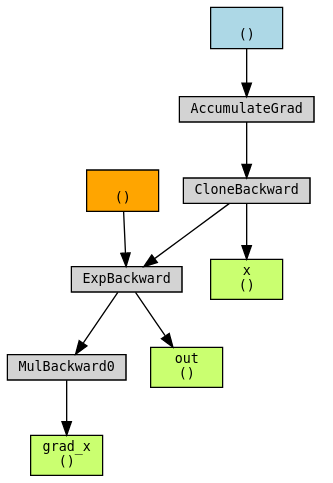## Saving Intermediate Results¶

A more tricky case is when we need to save an intermediate result. We demonstrate this case by implementing:

$sinh(x) := \frac{e^x - e^{-x}}{2}$

Since the derivative of sinh is cosh, it might be useful to reuse exp(x) and exp(-x), the two intermediate results in forward in the backward computation.

Intermediate results should not be directly saved and used in backward though. Because forward is performed in no-grad mode, if an intermediate result of the forward pass is used to compute gradients in the backward pass the backward graph of the gradients would not include the operations that computed the intermediate result. This leads to incorrect gradients.

class Sinh(torch.autograd.Function):
@staticmethod
def forward(ctx, x):
expx = torch.exp(x)
expnegx = torch.exp(-x)
ctx.save_for_backward(expx, expnegx)
# In order to be able to save the intermediate results, a trick is to
# include them as our outputs, so that the backward graph is constructed
return (expx - expnegx) / 2, expx, expnegx

@staticmethod
expx, expnegx = ctx.saved_tensors
# We cannot skip accumulating these even though we won't use the outputs
# directly. They will be used later in the second backward.

def sinh(x):
# Create a wrapper that only returns the first output
return Sinh.apply(x)

x = torch.rand(3, 3, requires_grad=True, dtype=torch.double)


Use torchviz to visualize the graph:

out = sinh(x)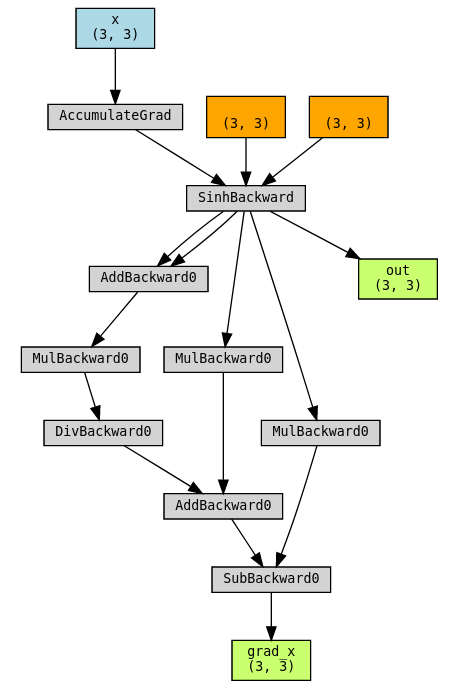## Saving Intermediate Results: What not to do¶

Now we show what happens when we don’t also return our intermediate results as outputs: grad_x would not even have a backward graph because it is purely a function exp and expnegx, which don’t require grad.

class SinhBad(torch.autograd.Function):
# This is an example of what NOT to do!
@staticmethod
def forward(ctx, x):
expx = torch.exp(x)
expnegx = torch.exp(-x)
ctx.expx = expx
ctx.expnegx = expnegx
return (expx - expnegx) / 2

@staticmethod
expx = ctx.expx
expnegx = ctx.expnegx


Use torchviz to visualize the graph. Notice that grad_x is not part of the graph!

out = SinhBad.apply(x)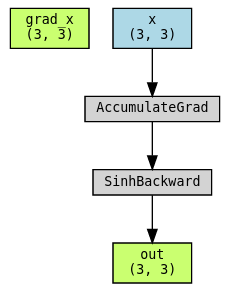## When Backward is not Tracked¶

Finally, let’s consider an example when it may not be possible for autograd to track gradients for a functions backward at all. We can imagine cube_backward to be a function that may require a non-PyTorch library like SciPy or NumPy, or written as a C++ extension. The workaround demonstrated here is to create another custom function CubeBackward where you also manually specify the backward of cube_backward!

def cube_forward(x):
return x**3

return grad_out * 3 * x**2

return grad_out * 3 * x**2

@staticmethod
def forward(ctx, x):
ctx.save_for_backward(x)
return cube_forward(x)

@staticmethod
x, = ctx.saved_tensors

@staticmethod

@staticmethod



Use torchviz to visualize the graph:

out = Cube.apply(x)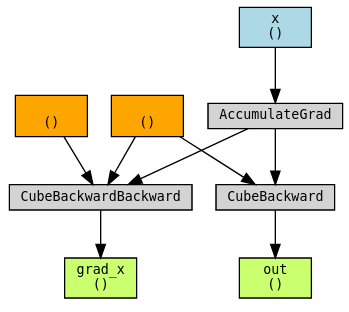To conclude, whether double backward works for your custom function simply depends on whether the backward pass can be tracked by autograd. With the first two examples we show situations where double backward works out of the box. With the third and fourth examples, we demonstrate techniques that enable a backward function to be tracked, when they otherwise would not be.

더 궁금하시거나 개선할 내용이 있으신가요? 커뮤니티에 참여해보세요!

이 튜토리얼이 어떠셨나요? 평가해주시면 이후 개선에 참고하겠습니다! :)

© Copyright 2018-2023, PyTorch & 파이토치 한국 사용자 모임(PyTorch Korea User Group).

Built with Sphinx using a theme provided by Read the Docs.

## PyTorchKorea @ GitHub

파이토치 한국 사용자 모임을 GitHub에서 만나보세요.

GitHub로 이동

## 한국어 튜토리얼

한국어로 번역 중인 PyTorch 튜토리얼입니다.

튜토리얼로 이동

## 커뮤니티

다른 사용자들과 의견을 나누고, 도와주세요!

커뮤니티로 이동